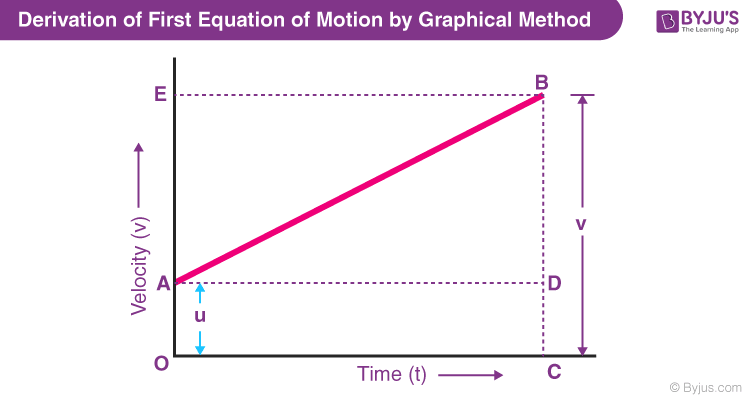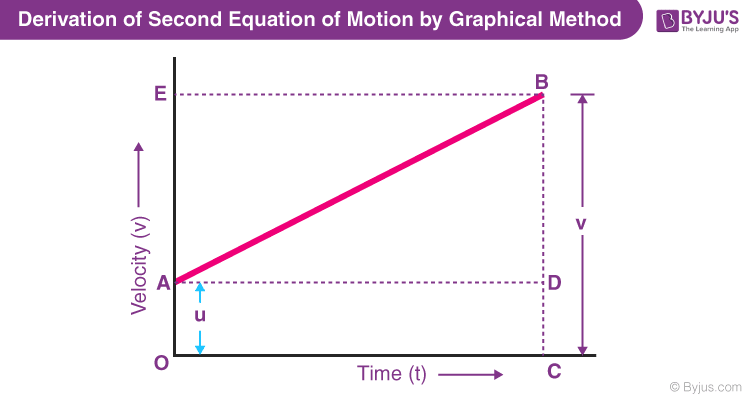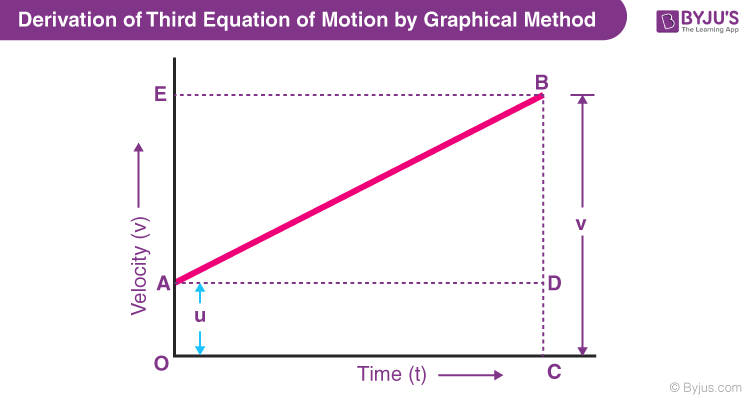Defend yourself better by mastering the science of immunity and vaccines. Secure your free spot, now! Defend yourself better by mastering the science of immunity and vaccines. Secure your free spot, now!

# Derivation of Equations of Motion

Equations of motion of kinematics describe the basic concept of the motion of an object such as the position, velocity or acceleration of an object at various times. These three equations of motion govern the motion of an object in 1D, 2D and 3D. The derivation of the equations of motion is one of the most important topics in Physics. In this article, we will show you how to derive the first, second and third equation of motion by graphical method, algebraic method and calculus method.

## Definition of Equations of Motion

Equations of motion, in physics, are defined as equations that describe the behaviour of a physical system in terms of its motion as a function of time.

There are three equations of motion that can be used to derive components such as displacement(s), velocity (initial and final), time(t) and acceleration(a). The following are the three equations of motion:

• First Equation of Motion :
$$\begin{array}{l}v=u+at\end{array}$$
• Second Equation of Motion :
$$\begin{array}{l}s=ut+\frac{1}{2}at^2\end{array}$$
• Third Equation of Motion :
$$\begin{array}{l}v^2=u^2+2as\end{array}$$

To brush up on the basics of motion, refer to the article listed below::

## Suggested Videos## Derivation of Equation of Motion

The equations of motion can be derived using the following methods:

• Derivation of equations of motion by Simple Algebraic Method
• Derivation of equations of Motion by Graphical Method
• Derivation of equations of Motion by Calculus Method

In the next few sections, the equations of motion are derived by all the three methods in a simple and easy to understand way.

## Derivation of First Equation of Motion

For the derivation, let us consider a body moving in a straight line with uniform acceleration. Then, let the initial velocity be u, acceleration is denoted as a, the time period is denoted as t, velocity is denoted as v, and the distance travelled is denoted as s.

### Derivation of First Equation of Motion by Algebraic Method

We know that the acceleration of the body is defined as the rate of change of velocity.

Mathematically, acceleration is represented as follows:

$$\begin{array}{l}a=\frac{v-u}{t}\end{array}$$

where v is the final velocity and u is the initial velocity.

Rearranging the above equation, we arrive at the first equation of motion as follows:

 $$\begin{array}{l}v=u+at\end{array}$$

### Derivation of First Equation of Motion by Graphical Method

The first equation of motion can be derived using a velocity-time graph for a moving object with an initial velocity of u, final velocity v, and acceleration a.In the above graph,

• The velocity of the body changes from A to B in time t at a uniform rate.
• BC is the final velocity and OC is the total time t.
• A perpendicular is drawn from B to OC, a parallel line is drawn from A to D, and another perpendicular is drawn from B to OE (represented by dotted lines).

The following details are obtained from the graph above:

The initial velocity of the body, u = OA

The final velocity of the body, v = BC

From the graph, we know that

BC = BD + DC

Therefore, v = BD + DC

v = BD + OA (since DC = OA)

Finally,

v = BD + u (since OA = u) (Equation 1)

Now, since the slope of a velocity-time graph is equal to acceleration a.

So,

a = slope of line AB

Since AD = AC = t, the above equation becomes:

BD = at (Equation 2)

Now, combining Equation 1 & 2, the following is obtained:

 v = u + at

### Derivation of First Equation of Motion by Calculus Method

Since acceleration is the rate of change of velocity, it can be mathematically written as:

$$\begin{array}{l}a=\frac{dv}{dt}\end{array}$$

Rearranging the above equation, we get

$$\begin{array}{l}adt=dv\end{array}$$

Integrating both the sides, we get

$$\begin{array}{l}\int_{0}^{t}adt=\int_{u}^{v}dv\end{array}$$
$$\begin{array}{l}at=v-u\end{array}$$

Rearranging, we get

 $$\begin{array}{l}v=u+at\end{array}$$

## Derivation of Second Equation of Motion

For the derivation of the second equation of motion, consider the same variables that were used for derivation of the first equation of motion.

### Derivation of Second Equation of Motion by Algebraic Method

Velocity is defined as the rate of change of displacement. This is mathematically represented as:

$$\begin{array}{l}Velocity=\frac{Displacement}{Time}\end{array}$$

Rearranging, we get

$$\begin{array}{l}{Displacement}=Velcoity\times Time\end{array}$$

If the velocity is not constant then in the above equation we can use average velocity in the place of velocity and rewrite the equation as follows:

$$\begin{array}{l}{Displacement}=(\frac{Initial\,Velocity + Final\,Velocity}{2})\times Time\end{array}$$

Substituting the above equations with the notations used in the derivation of the first equation of motion, we get

$$\begin{array}{l}s=\frac{u+v}{2}\times t\end{array}$$

From the first equation of motion, we know that v = u + at. Putting this value of v in the above equation, we get

$$\begin{array}{l}s=\frac{(u+(u+at))}{2}\times t\end{array}$$
$$\begin{array}{l}s=\frac{2u+at}{2}\times t\end{array}$$
$$\begin{array}{l}s=(\frac{2u}{2}+\frac{at}{2})\times t\end{array}$$
$$\begin{array}{l}s=(u+\frac{1}{2}at)\times t\end{array}$$

On further simplification, the equation becomes:

 $$\begin{array}{l}s=ut+\frac{1}{2}at^2\end{array}$$

## Derivation of Second Equation of Motion by Graphical MethodFrom the graph above, we can say that

Distance travelled (s) = Area of figure OABC = Area of rectangle OADC + Area of triangle ABD

$$\begin{array}{l}s=(\frac{1}{2}\times AD\times BD)+(OA\times OC)\end{array}$$

As OA=u and OC=t, the above equation becomes,

$$\begin{array}{l}s=(\frac{1}{2} \times AD \times BD)+(u\times t)\end{array}$$

As BD =at (from the graphical derivation of 1st equation of motion), the equation becomes,

$$\begin{array}{l}s=\frac{1}{2}\times t\times at+ut\end{array}$$

On further simplification, the equation becomes

 $$\begin{array}{l}s=ut+\frac{1}{2}at^2\end{array}$$

### Derivation of Second Equation of Motion by Calculus Method

Velocity is the rate of change of displacement.

Mathematically, this is expressed as

$$\begin{array}{l}v=\frac{ds}{dt}\end{array}$$

Rearranging the equation, we get

$$\begin{array}{l}ds=vdt\end{array}$$

Substituting the first equation of motion in the above equation, we get

$$\begin{array}{l}ds=(u+at)dt\end{array}$$
$$\begin{array}{l}ds=(u+at)dt=(udt+atdt)\end{array}$$

Integrating both sides, we get

$$\begin{array}{l}\int_{0}^{s}ds=\int_{0}^{t}udt+\int_{0}^{t}atdt\end{array}$$

On further simplification, the equations becomes:

 $$\begin{array}{l}s=ut+\frac{1}{2}at^2\end{array}$$

## Derivation of Third Equation of Motion

For the derivation of the third equation of motion, consider the same variables that were used for the derivation of the first and second equations of motion.

### Derivation of Third Equation of Motion by Algebraic Method

We know that displacement is the product of average velocity and time. Mathematically, this can be represented as:

$$\begin{array}{l}Displacement=(\frac{Initial\,Velocity+Final\,Velocity}{2})\times t\end{array}$$

Substituting the standard notations, the above equation becomes

$$\begin{array}{l}s=(\frac{u+v}{2})\times t\end{array}$$

From the first equation of motion, we know that

$$\begin{array}{l}v=u+at\end{array}$$

Rearranging the above formula, we get

$$\begin{array}{l}t=\frac{v-u}{a}\end{array}$$

Substituting the value of t in the displacement formula, we get

$$\begin{array}{l}s=(\frac{v+u}{2})(\frac{v-u}{a})\end{array}$$
$$\begin{array}{l}s=(\frac{v^2-u^2}{2a})\end{array}$$
$$\begin{array}{l}2as={v^2-u^2}\end{array}$$

Rearranging, we get

 $$\begin{array}{l}v^2=u^2+2as\end{array}$$

### Derivation of Third Equation of Motion by Graphical MethodFrom the graph, we can say that

The total distance travelled, s is given by the Area of trapezium OABC.

Hence,

s = ½ × (Sum of Parallel Sides) × Height

s = 1/2 x (OA + CB) x OC

Since, OA = u, CB = v, and OC = t

The above equation becomes

s = 1/2 x (u+v) x t

Now, since t = (v – u)/ a

The above equation can be written as:

s = ½ x ((u+v) × (v-u))/a

Rearranging the equation, we get

s = ½ x (v+u) × (v-u)/a

s = (v2-u2)/2a

Third equation of motion is obtained by solving the above equation:

 v2 = u2+2aS

### Derivation of Third Equation of Motion by Calculus Method

We know that acceleration is the rate of change of velocity and can be represented as:

$$\begin{array}{l}a=\frac{dv}{dt}…. (1)\end{array}$$

We also know that velocity is the rate of change of displacement and can be represented as:

$$\begin{array}{l}v=\frac{ds}{dt}…. (2)\end{array}$$

Cross multiplying (1) and (2), we get

$$\begin{array}{l}a\frac{ds}{dt}=v\frac{dv}{dt}\end{array}$$
$$\begin{array}{l}\int_{0}^{s}ads=\int_{u}^{v}vdv\end{array}$$
$$\begin{array}{l}as=\frac{v^2-u^2}{2}\end{array}$$
 $$\begin{array}{l}v^2=u^2+2as\end{array}$$

This is how we derive the three equations of motion by algebraic, graphical and calculus method.

#### Important derivations and formulae of Class 12 Physics#### Top 10 NTSE Important Questions on Motion Class 9Stay tuned to BYJU’S and Fall in Love with Learning!

Test your knowledge on Derivation of equation of motion

1. it is a very good app for learning

2. it is very useful for me!

3. Very good for learning physic preparation

4. It is nice app

• Really it is good app

5. It is a nice app

6. NICE APP

7. the best app for learning and visualising ourselves

8. This is helpful! Thank you.

9. hi ! thank u so much ❤❤ this is helpful

10. Thanks

11. nice app for learning

12. thanks

13. Best app for learning and visualising concepts

14. I will never forget any of these derivations.